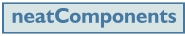Search:
 Previous page Data Integration Next page Expressions - Function ReferenceExpressions can contain the following functions.

Functions can be combined using the Operators, and can be nested.

Functions are grouped in the following categories:

## Conditional

### COALESCE

COALESCE(value1, value2[, value10])

Returns the first non-null value. Can be used as a 'NullToZero' function if the second parameter is 0.

### IIF

IIF(condition, truevalue, falsevalue)

If [condition] evaluates to true, null, return [truevalue] otherwise return [falsevalue]

## Date / Time

### DATEDIFF

DATEDIFF(datetime1, datetime2)

Returns [datetime1] - [datetime2] in days

### DATE_FORMAT

DATE_FORMAT(datetime, format)

Formats [datetime] according to [format]

### DAY

DAY(datetime)

Day of month of [datetime]  (1-31)

### HOUR

HOUR(datetime)

Hour of [datetime] (0-23)

### INTERVAL

INTERVAL(integer,[YEAR|MONTH|DAY|HOUR|MINUTE|SECOND])

Returns an interval that can be added to / subtracted from a date / time

Note: In an expression the Interval should be placed after the date ie Date + Interval, not Interval + Date

### MINUTE

MINUTE(datetime)

Minute of [datetime]   (0-59)

### MONTH

MONTH(datetime)

Month of [datetime]   (1-12)

### NOW

NOW()

Current date and time

### SECOND

SECOND(datetime)

Second of [datetime]  (0-59)

### UTC_TIMESTAMP

UTC_TIMESTAMP()

Current UTC date and time

## Math

ABS(number)

Absolute value

ACOS(number)

Inverse cosine

ASIN(number)

Inverse sine

ATAN(number)

Inverse tangent

### CEIL

CEIL(number)

Smallest integer not less than argument

COS(number)

Cosine

COT(number)

Cotangent

DEGREES(number)

EXP(number)

Exponential

### FLOOR

FLOOR(number)

Largest integer less than argument

### LN

LN(number)

Natural logarithm

### LOG

LOG(number1, number2)

Logarithm to base [number2]

### MOD

MOD(number1, number2)

Remainder of [number1] / [number2]

PI()

Pi constant

### POWER

POWER(number1, number2)

[number1] raised to the power [number2]

### ROUND

ROUND(number, precision)

Round to [precision] decimal places

### SIGN

SIGN(number)

Sign of the argument (-1, 0, 1)

SIN(number)

Sine

SQRT(number)

Square root

TAN(number)

Tangent

## Text

### CONST.CURRENTSITE.URL

CONST.CURRENTSITE.URL()

Returns the domain name of the site. If the site is listening on multiple domain names, it will return the domain name that is being used by this particular request.

### LEFT

LEFT(text, length)

Return first [length] characters in the string

### LOWER

LOWER(text)

Convert text to lower case

LTRIM(text)

### REPEAT

REPEAT(text, number)

Repeat [text] the specified [number] times

### REPLACE

REPLACE (string,find,replace)

Replaces instances in the [string] of the [find] text with the [replace] text

### REVERSE

REVERSE(text)

Return reversed string

### RIGHT

RIGHT(text, length)

Return last [length] characters in the string

### RTRIM

RTRIM(text)

Remove trailing spaces

### SUBSTR

SUBSTR(text, start[, length])

Return [length] characters from character [start] in the string

TRIM(text)

### UPPER

UPPER(text)

Convert text to upper case

Extended use

Conditional expressions can be extended by combination.

For example, DATEDIFF only calculates the difference between two date-times in whole numbers of days. If you need to calculate the difference in a number of hours you can use DATEDIFF in combination with the HOUR() function as follows:

(DATEDIFF([t:hour difference]![finish time], [t:hour difference]![start time]) * 24) + (HOUR([t:hour difference]![finish time]) - HOUR([t:hour difference]![start time]))Managing data